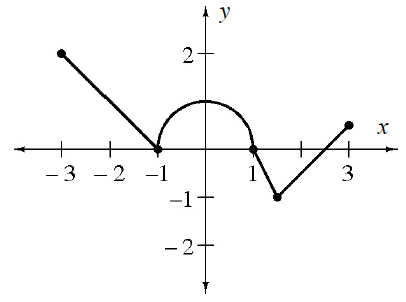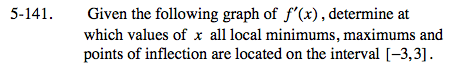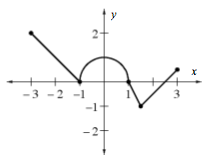### Home > CALC > Chapter 5 > Lesson 5.4.1 > Problem5-141

5-141.
1. Given the graph of f ′(x) below, determine the values of x for which f (x) has local minima, maxima, and points of inflection on the interval [−3, 3]. Homework Help ✎

2.You are looking at the graph of f '(x), but you are being asked to describe the graph of f(x).A minimum value on f(x) is where the y-values change from decreasing to increasing. How does that show up on the f '(x) graph?

Local minima on f(x) are located anywhere that the graph of f '(x) changes from negative to positive. Local maxima are the reverse.

Points of inflection on f(x) occur where f '(x) changes the sign of its slope. This happens twice.